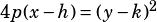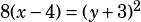Subject Test Question:

The standard equation of a parabola with focus (2, -3) and directrix x=6 is

B) x-4 = -8(y+3)^2.

I did not get this answer. Instead I got (y+3)^2 = -8(x-4)

Purplemath has a great review of this stuff that you may find helpful.

Here are the things you must know:

• The “standard equation” they’re looking for is in this form:.
• The parabola’s vertex is always halfway between the focus and the directrix, so it will be at (4, –3).
• A parabola opens away from its directrix, which is a vertical line, so this is a sideways parabola opening left.
• Since the vertex of the parabola is 2 units from the directrix, p = 2.

Take the vertex of (4, –3) and your calculated p value and put them into the equation: